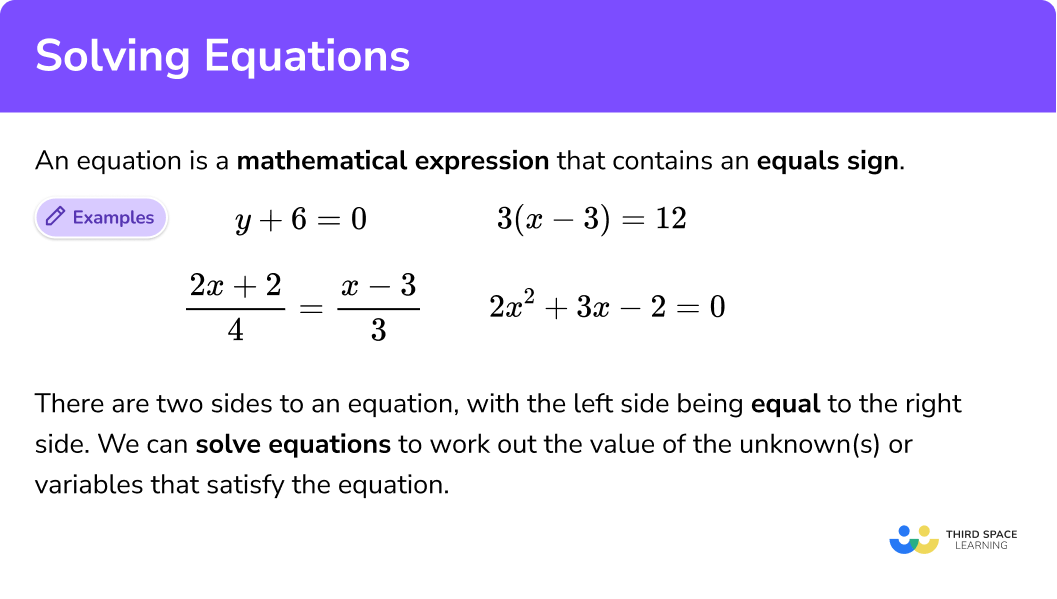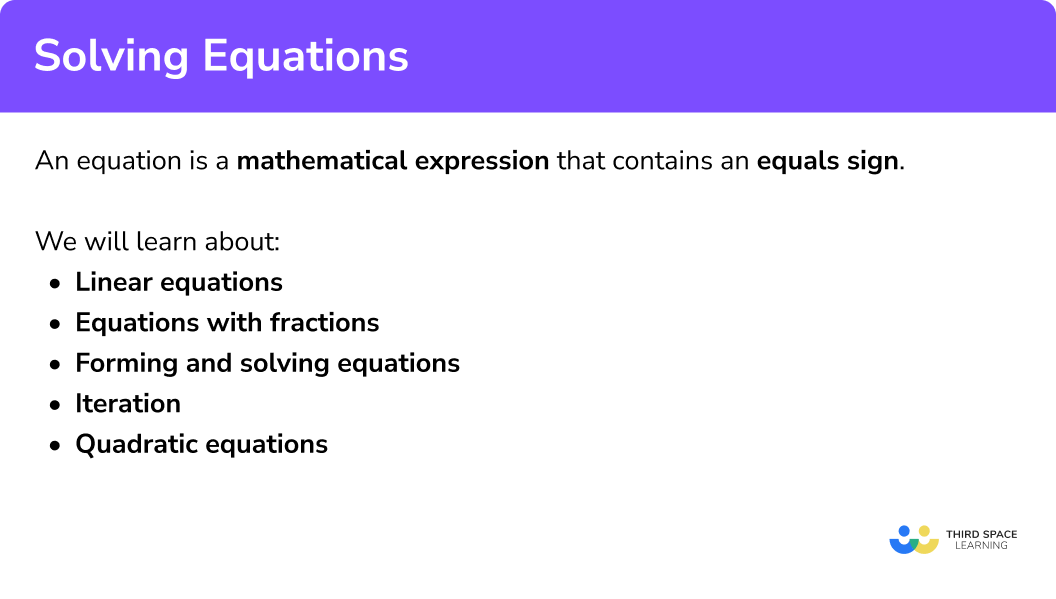GCSE Maths Algebra Equations

Solving Equations

# Solving Equations

Here we break down everything you need to know about solving equations. You’ll learn what linear and quadratic algebraic equations are, and how to solve all the different types of them.

At the end you’ll find solving equations worksheets based on Edexcel, AQA and OCR exam questions, along with further guidance on where to go next if you’re still stuck.

## What is an equation?

An equation is a mathematical expression that contains an equals sign.

E.g.

\begin{aligned} y+6&=0\\ 3(x-3)&=12\\ \frac{2x+2}{4}&=\frac{x-3}{3}\\ 2x^{2}+3x-2&=0 \end{aligned}

There are two sides to an equation, with the left side being equal to the right side.

Equations will often involve algebra and contain unknowns (variables) which we often represent with letters such as x or y.

We can solve simple equations and more complicated equations to work out the value of these unknowns; they could involve fractions, decimals or integers.

Step-by-step guide: Forming and solving equations

### What is an equation?## How to solve equations

In order to solve equations, we need to work out the value of the unknown variable by adding, subtracting, multiplying or dividing both sides of the equation by the same value.

In GCSE Maths there are two main types of equations that we need to solve, both of which are covered below.

### Explain how to solve equations## Solving equations methods

Within solving equations, you will find lessons on linear equations and quadratic equations.

Each method of solving equations is summarised below. For detailed examples, practice questions and worksheets on each one follow the links to the step by step guides.

### 1. Linear equations

There are 5 main types of linear equations we can solve.

Example of solving an equation with:

1. One unknown

2Unknown on both sides

3With brackets

4With fractions

Step-by-step guide: Equations with fractions

5Powers (exponents) and roots

We can check that our solution is correct by substituting it into the original equation.

Step-by-step guide: Linear equations

Step-by-step guide: Iteration maths

There are 4 main ways to solve quadratic equations.

Example of solving a quadratic equation by:

1. Factorising

Step-by-step guide: Solving quadratic equations by factorising

\begin{aligned} x^{2}+4x-5&=0\\ \\ x&=\frac{-b\pm\sqrt{b^2-4ac}}{2a}\\ \\ a&=1, \quad b=4, \quad c=-5\\ x&=\frac{-4\pm\sqrt{4^2-4(1)(-5)}}{2(1)}\\ \\ x&=1, \qquad x=-5\\ \end{aligned}

3Complete the square

\begin{aligned} x^{2}+4x-5&=0\\ \\ (x+2)^{2}-9&=0\\ (x+2)^{2}&=9\\ x+2&=\pm\sqrt{9}\\ \\ x&=3-2\\ &=1\\ \\ x&=-3-2\\ &=-5 \end{aligned}

Step-by-step guide: Completing the square

4Graphically

$x^{2}+4x-5=0$

The solutions/roots are found when the graph equals 0 (crosses the x-axis).

$x=1,\qquad x=-5$

We can check that our solution is correct by substituting it into the original equation.

Step-by-step guide: Solving quadratic equations graphically

## Practice solving equations questions

1. Solve: 4x-2=14

x=3x=4x=14x=3.54x-2=14

4x=16

Divide both sides by   4

x=4

2. Solve: 3x-8=x+6

x=7x=4x=8x=63x-8=x+6

3x=x+14

Subtract x from both sides

2x=14

Divide both sides by 2

x=7

3. Solve: 3(x+3)=2(x-2)

x=2x=-3x=-13x=133(x+3)=2(x-2)

Expanding the brackets

3x+9=2x-4

Subtract 9 from both sides

3x=2x-13

Subtract 2x from both sides

x=-13

4. Solve: \frac{2 x+2}{3}=\frac{x-3}{2}

x=2x=-3x=-13x=13\frac{2 x+2}{3}=\frac{x-3}{2}

Multiply by 6 (the lowest common denominator) and simplify

2(2x+2)=3(x-3)

Expand the brackets

4x+4=3x-9

Subtract 4 from both sides

4x=3x-13

Subtract 3x from both sides

x=-13

5. Solve: \frac{3 x^{2}}{2}=24

x=\pm 4x=\pm 2x=\pm 3x=\pm 6\frac{3 x^{2}}{2}=24

Multiply both sides by 2

3 x^{2}=48

Divide both sides by 3

x^{2}=16

Square root both sides

x=\pm 4

6. Solve by factorising: x^{2}-13 x+30=0x^{2}-13 x+30=0

Factorise as a double bracket

(x-3)(x-10)=0

Set each bracket equal to zero and solve, hence

x=3 or x=10

7. Solve by factorising: 2 x^{2}+3 x-20=02 x^{2}+3 x-20=0

Factorise as a double bracket

(2x-5)(x+4)

Set each bracket equal to zero and solve, hence

x=\frac{5}{2}, \; x=-4

8. (H) Solve by using the quadratic formula:  x^{2}-4 x-3=0

x=-4.65 \; (3.5 . f), \quad x=-0.646 \;(3.s.f)x=4.65 \; (3.5 . f), \quad x=0.646 \; (3.s.f)x=4.65 \; (3.5 . f), \quad x=-0.646 \; (3.s.f)x=-4.65 \; (3.5 . f), \quad x=0.646 \; (3.s.f)Substitution into the quadratic formula gives

9. (H) Solve by using the quadratic formula:  2 x^{2}+4 x-4=0

x=0.732 \; (3.s.f),\quad x=-2.73 \; (3.s.f)x=-0.732 \; (3.s.f),\quad x=-2.73 \; (3.s.f)x=0.732 \; (3.s.f),\quad x=2.73 \; (3.s.f)x=-0.732 \; (3.s.f),\quad x=2.73 \; (3.s.f)Substitution into the quadratic formula gives

10. (H) Solve by completing the square: x^{2}-6 x+5=0Completing the square

(x-3)^{2}-4=0

(x-3)^{2}=4

Square root both sides

x-3=\pm 2

x=3\pm 2

So x=5, \; x=1

## Solving equations GCSE questions

1. Solve: 4y = 36

y = 9

(1 mark)

2. Solve: x2 − 5x − 24 = 0

x = − 3, x = 8

(3 marks)

3. Solve: 7y − 8 = 13

y = 3

(2 marks)

## Learning checklist

• Use algebraic methods to solve linear equations
• Solve quadratic equations algebraically by factorising
• Solve quadratic equations algebraically by completing the square (H)